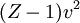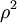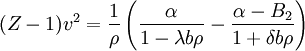Linear isothermal regularityThis article is a 'stub' page, it has no, or next to no, content. It is here at the moment to help form part of the structure of SklogWiki. If you add sufficient material to this article then please remove the {{Stub-general}} template from this page.
The linear isothermal regularity was discovered by Parsafar in 1991. It suggests that$(Z-1)v^2$ vs$\rho^2$ is linear. This led to a new Van der Waals like equation of state (Ref. 2 Eq. 8):$(Z-1) v^2 = \frac{1}{\rho } \left( \frac{\alpha}{1-\lambda b \rho} - \frac{\alpha - B_2}{1+ \delta b \rho} \right)$